【PHP】当mysql遇上PHP

+关注继续查看

博客提纲

• 利用PHP连接mySQL数据库
• 两套接口：面向对象和面向过程
• 实现写改删查（CUBD）实例
• 通过prepare语句处理相同类型的不同SQL语句
• 通过bind_param()绑定参数,及相关注意事项
• 通过bind_result()绑定结果，以及相关注意事项
• 将文本写入数据库前应做的检测和处理
• 检查是否为空（未输入值）
• 去首尾空格
• 魔鬼字符串转义

一.利用PHP连接mySQL数据库PHP河的河神问他。。。。PHP针对mysql数据库的操作有两套接口：面向对象接口和面向过程接口

• 面向对象接口：通过调用对象中的函数完成数据库操作
• 面向过程接口：直接调用PHP内置的函数实现数据库操作

读操作：

面向对象：

<?php
@$mysqli = new mysqli('localhost', 'root', 'phw441423', 'penghuwan');//(主机，账号，密码，数据库) 返回一个mysqli对象 if($mysqli->connect_error){//当有连接错误的时候，结束脚本运行并且报错
die('连接错误，这个错误是'.$mysqli->connect_error);//die()函数：1结束脚本运行，2输出一段文本（括号内） }$query = "SELECT * FROM mytable";//把一段SQL语句保存在$query变量中$mysqli_result = $mysqli->query($query);//通过调用上面返回的mysqli对象中的方法，返回一个结果集对象（mysqli_result）
while($row =$mysqli_result->fetch_assoc()){//调用mysqli_result的方法fetch_assoc()后，返回的是一个数组变量$row echo$row['name'];//访问返回数组变量$row中的数组成员，对应mytable表中的name列 echo$row['number'];;//访问返回数组变量$row中的数组成员，对应mytable表中的number echo "<br/>"; }$mysqli_result->free();//释放结果集
$mysqli ->close();//关闭数据库连接 ?> 首先通过 new mysqli($host, $username,$passwd, $dbname) 获取一个mysqli对象，然后在下面我们就可以通过调用对象中的方法query方法去实现写改删查 运行结果思维导图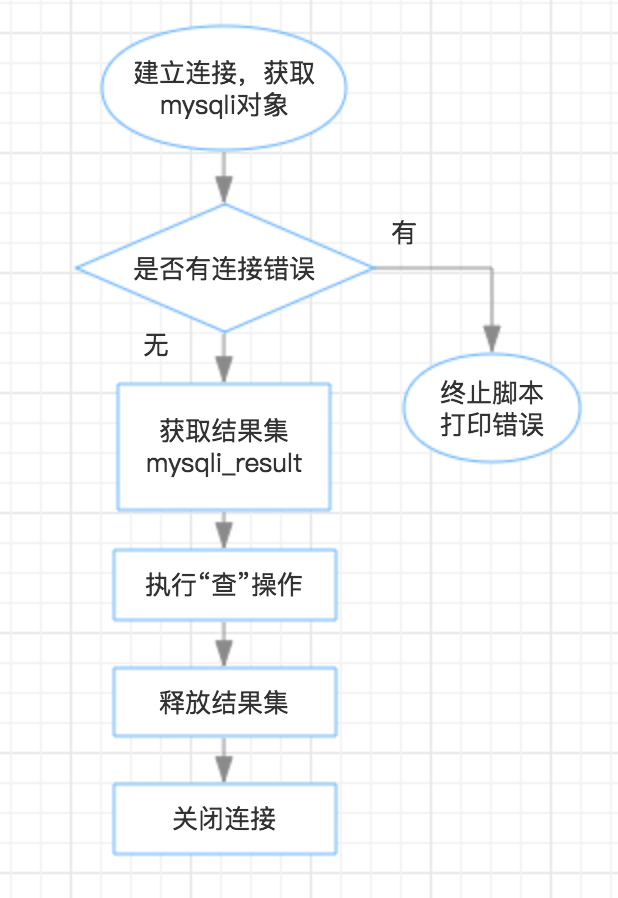上面的例子中，一个关键的方法是mysqli对象query方法，意为查询.但实际上，它除了能运行“查”的SQL语句外，还能运行“写改删”的SQL语句。 关于query的返回值: • 执行失败，返回false • 执行成功 • 如果执行的语句，即query是SELECT，SHOW，EXPLAIN 或 DESCRIBE，则返回一个结果集对象 • 如果是其他，则返回false 面向过程： <?php @$mysqli = mysqli_connect('localhost', 'root', 'phw441423', 'penghuwan');//(主机，账号，密码，数据库) 返回一个mysqli对象
if(mysqli_connect_error()){//当有连接错误的时候，结束脚本运行并且报错
die('连接错误，这个错误是'.mysqli_connect_error());;//die()函数：1结束脚本运行，2输出一段文本（括号内）
}
$query = "SELECT * FROM mytable";//把一段SQL语句保存在$query变量中
$mysqli_result = mysqli_query($mysqli, $query);//在面向过程风格里，$mysqli对象成了该方法中的参数，也返回一个结果集对象（mysqli_result）
while($row = mysqli_fetch_assoc($mysqli_result)){// 返回的是一个数组变量$row echo$row['name'];//访问返回数组变量$row中的数组成员，对应mytable表中的name列 echo$row['number'];;//访问返回数组变量$row中的数组成员，对应mytable表中的number echo "<br/>"; } mysqli_free_result($mysqli_result);//释放结果集
mysqli_close($mysqli);//关闭数据库连接 ?> 【注意点】 1. mysqli_fetch_assoc（面向过程）和fetch_assoc（面向对象）这两个方法返回的是一个关联数组变量$row
2. 在命令行界面里，我们需要做选择数据库的选择，即使用“USE 所选数据库”这个命令，但在这里我们在一开始连接的时候就选择了数据库了。例如：mysqli_connect('localhost', 'root', 'phw441423', 'penghuwan')；中我们选择了数据库penghuwan所以就不用写USE语句了
3. 最后记得要释放结果集和关闭连接

• 如何记忆？
一般情况下：面向过程函数名= mysqli_ +面向对象函数名
例如：
返回结果集对象的方法：
面向对象：query 面向过程：mysqli_query
从结果集对象中返回某一行（形式为关联数组）的方法：
面向对象：fetch_assoc 面向过程：mysqli_fetch_assoc

• 两者联系
一般情况下，面向对象接口中的对象将会成为面向过程接口中的第一个参数
例如：
通过mysqli对象取得结果集的时候：
面向过程：$mysqli_result = mysqli_query($mysqli, $query); 面向对象：$mysqli_result = $mysqli->query($query);

写操作：

面向对象：

<?php
@$mysqli = new mysqli('localhost', 'root', 'phw441423', 'penghuwan');//(主机，账号，密码，数据库) 返回一个mysqli对象 if($mysqli->connect_error){//当有连接错误的时候，结束脚本运行并且报错
die('连接错误，这个错误是'.$mysqli->connect_error);//die()函数：1结束脚本运行，2输出一段文本（括号内） }$query = "INSERT INTO mytable VALUES('C',30)";//把一段SQL语句保存在$query变量中$mysqli->query($query);// 此时返回的不是结果集对象，而是一个boolean，代表成功或失败$mysqli ->close();//关闭数据库连接
?>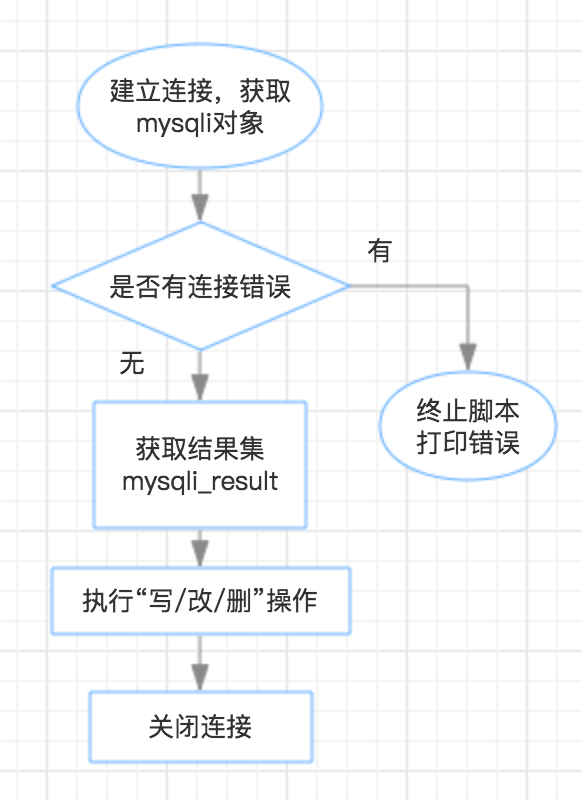二.通过prepare语句处理相同类型的不同SQL语句

通过bind_param()绑定参数,及相关注意事项

"SELECT * FROM mytable WHERE name = ‘A’"

"SELECT * FROM mytable WHERE name = ‘B’"D 40
E 50
<?php
@$mysqli = new mysqli('localhost', 'root', 'phw441423', 'penghuwan');//(主机，账号，密码，数据库) 返回一个mysqli对象 if($mysqli->connect_error){//当有连接错误的时候，结束脚本运行并且报错
die('连接错误，这个错误是'.$mysqli->connect_error);//die()函数：1结束脚本运行，2输出一段文本（括号内） }$query = "INSERT INTO mytable VALUES(?,?)";//,“?”表示模板中要被实际替换的变量
$stmt =$mysqli->prepare($query);//通过prepare函数生成mysqli_statement对象$name1 = 'D';
$number1 = 40;$stmt->bind_param("si",$name1,$number1);//通过mysqli_statement对象的bind_param方法用实际的变量替换模板中的"?"
$stmt->execute();//第一次执行$name2 = 'E';
$number2 = 50;$stmt->bind_param("si",$name2,$number2);//通过mysqli_statement对象的bind_param方法用实际的变量替换模板中的"?"
$stmt->execute();//第二次执行$stmt->close();//关闭mysqli_statement
$mysqli ->close();//关闭数据库连接 ?> 思维导图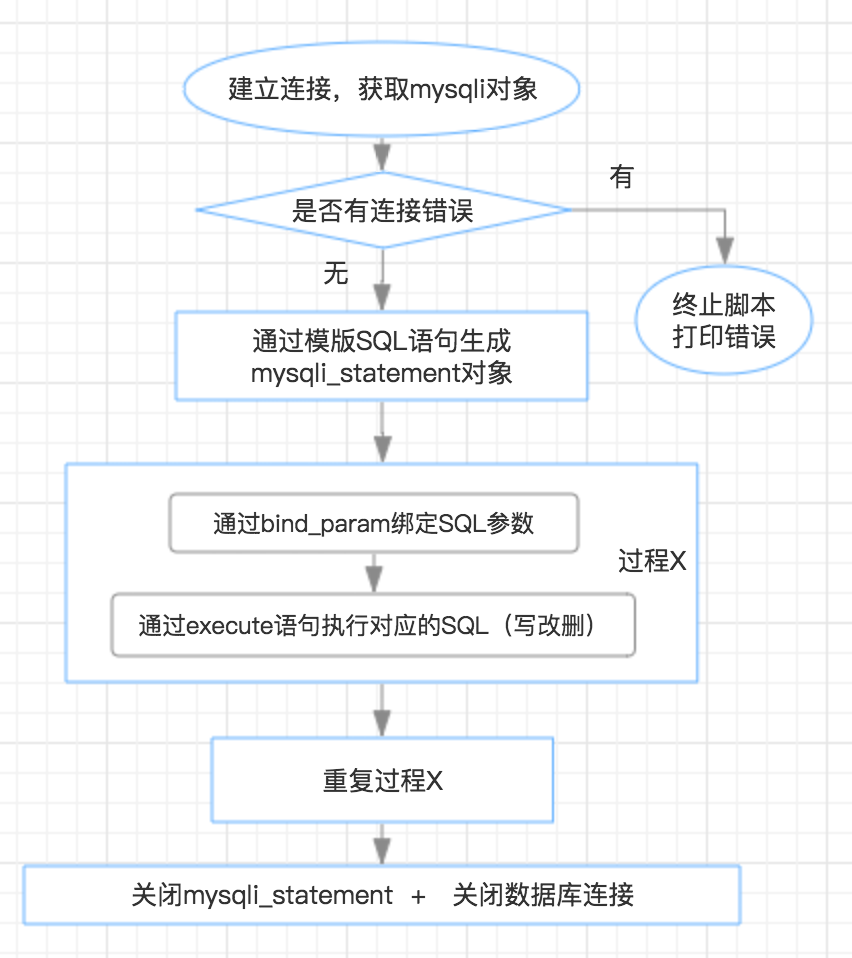运行结束后关键的一个方法是bind_param（）方法，它接受多个参数，其中 第一个参数代表后面参数的类型。 第一个参数是一个字符串，由固定顺序的字符组成，这些字符包括“s”,”i”,”d”,”b”,分别表示字符串，整型，双精度和二进制文本，依次代表后面参数的类型。 字符 代表类型 “s” 字符串 “i” 整型 “d” 双精度 “b” 二进制文本 例如：我们上面的$stmt->bind_param("si",$name1,$number1);代表：$name1是字符串类型，,$number1是整型

【注意】

• 不能直接向bind_param()第二个即以后的参数中写入具体的变量值！否则会报错：
例如，我们把：
<?PHP
$name1 = 'D';$number1 = 40;
$stmt->bind_param("si",$name1,$number1); ?> 改成： <?PHP$stmt->bind_param("si",'D',40);
?>【注意】

• 你只能写入变量的名称而不能写具体的类型值——
一个bind_param（）函数对应一个execute()函数，如果连续写多个bind_param（）再写execute()函数，相当于最后一个bind_param（）覆盖前面写的的 bind_param（）

$name1 = 'D';$number1 = 40;
$stmt->bind_param("si",$name1,$number1);//通过mysqli_statement对象的bind_param方法用实际的变量替换模板中的"?"$stmt->execute();//第一次执行

$name2 = 'E';$number2 = 50;
$stmt->bind_param("si",$name2,$number2);//通过mysqli_statement对象的bind_param方法用实际的变量替换模板中的"?"$stmt->execute();//第二次执行

$name1 = 'D';$number1 = 40;
$stmt->bind_param("si",$name1,$number1);//通过mysqli_statement对象的bind_param方法用实际的变量替换模板中的"?"$name2 = 'E';
$number2 = 50;$stmt->bind_param("si",$name2,$number2);//通过mysqli_statement对象的bind_param方法用实际的变量替换模板中的"?"
$stmt->execute();//第二次执行 运行结果：它并不会批量执行$name1,$number1和$name2,$number2的插入，而是只插入了$name2,$number2，因为最后一个bind_param（）覆盖前面写的的 bind_param（） 通过bind_result()绑定结果,及相关注意事项 上面的例子中我们演示了如何绑定参数，下面我来演示如何绑定结果，这里将用到bind_result（）函数： <?php @$mysqli = new mysqli('localhost', 'root', 'phw441423', 'penghuwan');//(主机，账号，密码，数据库) 返回一个mysqli对象
if($mysqli->connect_error){//当有连接错误的时候，结束脚本运行并且报错 die('连接错误，这个错误是'.$mysqli->connect_error);//die()函数：1结束脚本运行，2输出一段文本（括号内）
}
$query = "SELECT * FROM mytable";//prepare函数的参数$stmt = $mysqli->prepare($query);//通过prepare函数生成mysqli_statement对象
$stmt->bind_result($name,$number);//将执行结果绑定到$name和,$number中$stmt->execute();// 执行生成查询结果
while($stmt->fetch()){// 将查询结果中的第一行的列值分别赋给$name和,$number，同时游标移到下一行 echo$name.'   '.$number;//输出mytable中当前行各个列的列值 echo "<br/>"; }$stmt->close();//关闭mysqli_statement
$mysqli ->close();//关闭数据库连接 ?> 思维导图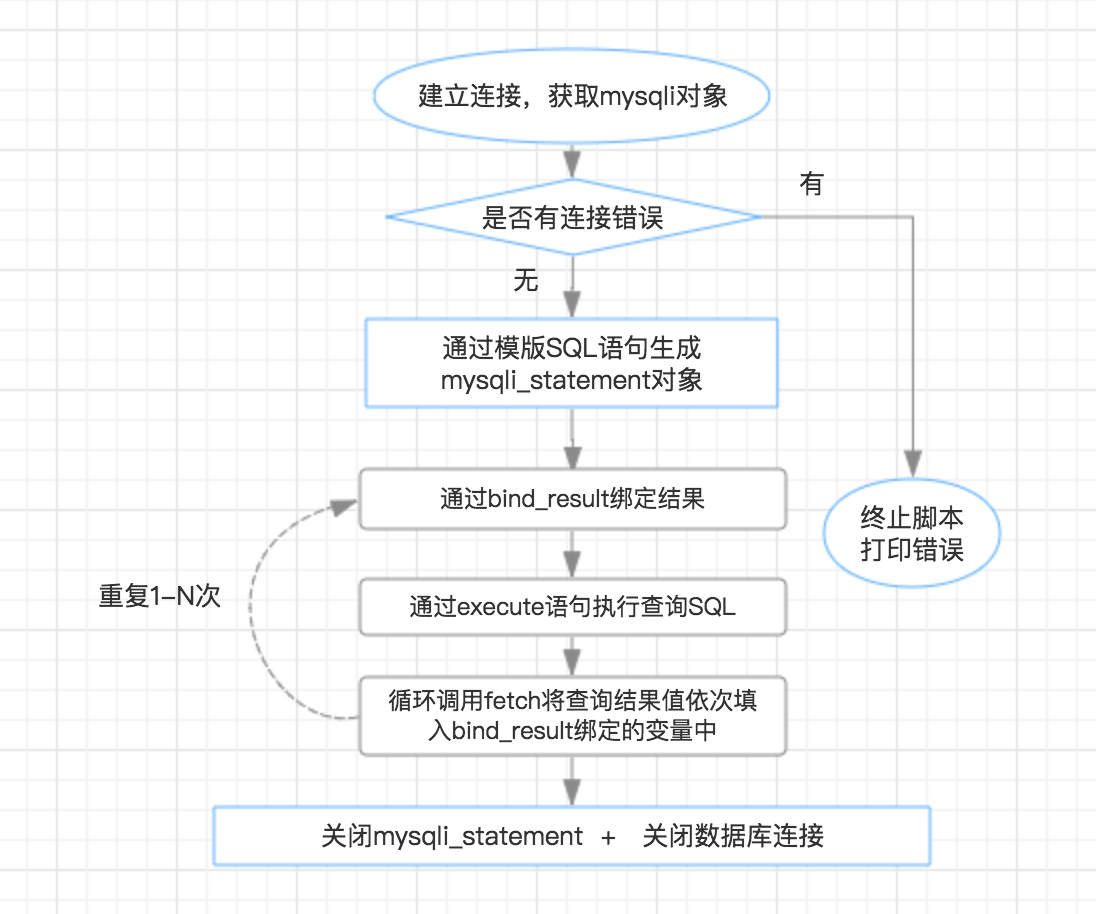运行结果如下：【注意】 • bind_param必须放在execute语句的前面，但bind_result放在execute前后均可 例如：我们将上面对应的代码改成：$stmt->execute();// 执行生成查询结果
$stmt->bind_result($name,$number);//将执行结果绑定到$name和,$number中 运行结果同上（但注意bind_result应放在fetch语句前） • excute（）执行完毕的时候，$name,$number仍为空，直到fetch（）第一次执行的时候，$name,$number才取到对应行的列值 将上面例子中对应代码改成：$stmt->bind_result($name,$number);//将执行结果绑定到$name和,$number中
$stmt->execute();// 执行生成查询结果 echo 'execute执行后$name的值为';
var_dump($name); 运行结果：• 同一个prepare模板可多次使用，但前后使用两个prepare模板中间，必须关闭现有的mysqli_statement$query1 = "SELECT name FROM mytable";
$stmt =$mysqli->prepare($query1);$stmt->execute();//执行第一个prepare模板语句

$query2 = "SELECT number FROM mytable";//prepare函数的参数$stmt = $mysqli->prepare($query2);
$stmt->execute();//执行第二个prepare模板语句 [注]，这就是24行提示的错误是，我对一个boolean值调用了execute函数 我尝试输出$stmt(最下面那个)，输出为false（这里不做展示了）

$stmt->close()： 即： <?PHP$query1 = "SELECT name FROM mytable";
$stmt =$mysqli->prepare($query1);$stmt->execute();//执行第一个prepare模板语句

$stmt->close();$query2 = "SELECT number FROM mytable";//prepare函数的参数
$stmt =$mysqli->prepare($query2);$stmt->execute();//执行第二个prepare模板语句
?>

三.将字符串写入数据库前应做的检测和处理

1. 检查输入是否为空值，这点就不加赘述了
2. 去除首尾空格（假设我们在录入数据库前没有去除空格的话，例如将“【空格】彭湖湾”录入数据库，那么在进行“【空格】彭湖湾”===“彭湖湾”的匹配时便会返回false）
3. 对魔术字符串转义（如果不进行转义，字符串中的双引号和单引号会对我们的SQL语句造成干扰）
<?php
$text =$_GET['text'];// 从from表单中name属性为“text”的输入框中取得值
if(!$text){//如果text为空则输出警告，并结束脚本 echo '您还没有输入任何值哦'; exit(); }$text = trim($text);//去除首尾空格 if(!get_magic_quotes_gpc()){//检查是否自动开启了魔术字符串转义，如果没有，则手动转义魔术字符串$text = addslashes($text); } echo '经过处理后的值'.$text;
echo "<br/>";
echo '重新取出值'.stripslashes($text); ?> 输入空值的时候：输入带空格和魔术字符串的文本——“【空格】penghuwan”参考资料 《php和mysql的web开发》--(澳)威利,(澳)汤姆森 著 PHP官方文档 链接：http://php.net/manual/zh/ stackOverFlow社区 链接: https://stackoverflow.com/其实啊，我只是把你们喝咖啡的时间，都用来喝啤酒而已 版权声明：本文内容由阿里云实名注册用户自发贡献，版权归原作者所有，阿里云开发者社区不拥有其著作权，亦不承担相应法律责任。具体规则请查看《阿里云开发者社区用户服务协议》和《阿里云开发者社区知识产权保护指引》。如果您发现本社区中有涉嫌抄袭的内容，填写侵权投诉表单进行举报，一经查实，本社区将立刻删除涉嫌侵权内容。 作者高分内容 更多 相关文章阿里云服务器怎么设置密码？怎么停机？怎么重启服务器？ 如果在创建实例时没有设置密码，或者密码丢失，您可以在控制台上重新设置实例的登录密码。本文仅描述如何在 ECS 管理控制台上修改实例登录密码。 10089 0使用NAT网关轻松为单台云服务器设置多个公网IP 在应用中，有时会遇到用户询问如何使单台云服务器具备多个公网IP的问题。 具体如何操作呢，有了NAT网关这个也不是难题。 26793 0阿里云服务器如何登录？阿里云服务器的三种登录方法 14500 0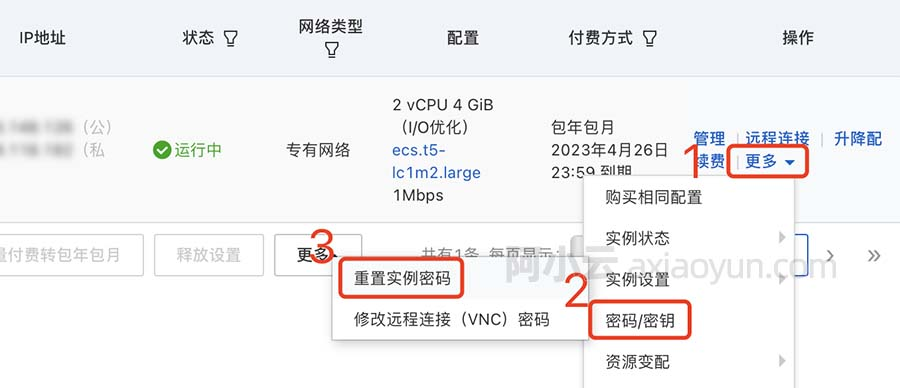阿里云服务器ECS远程登录用户名密码查询方法 阿里云服务器ECS远程连接登录输入用户名和密码，阿里云没有默认密码，如果购买时没设置需要先重置实例密码，Windows用户名是administrator，Linux账号是root，阿小云来详细说下阿里云服务器远程登录连接用户名和密码查询方法 11631 0Xshell使用SSH远程登录阿里云ECS服务器CentOS7 13112 0windows server 2008阿里云ECS服务器安全设置 最近我们Sinesafe安全公司在为客户使用阿里云ecs服务器做安全的过程中，发现服务器基础安全性都没有做。为了为站长们提供更加有效的安全基础解决方案，我们Sinesafe将对阿里云服务器win2008 系统进行基础安全部署实战过程！ 比较重要的几部分 1. 9161 0阿里云服务器如何登录？阿里云服务器的三种登录方法 购买阿里云ECS云服务器后如何登录？场景不同，阿里云优惠总结大概有三种登录方式： 登录到ECS云服务器控制台 在ECS云服务器控制台用户可以更改密码、更换系. 13891 0腾讯云服务器 设置ngxin + fastdfs +tomcat 开机自启动 在tomcat中新建一个可以启动的 .sh 脚本文件 /usr/local/tomcat7/bin/ export JAVA_HOME=/usr/local/java/jdk7 export PATH=$JAVA_HOME/bin/:\$PATH export CLASSPATH=.
4660 022405 054769 0

53

0

《2021云上架构与运维峰会演讲合集》

《零基础HTML入门教程》

《零基础CSS入门教程》